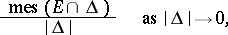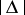# Density of a set

(diff) ← Older revision | Latest revision (diff) | Newer revision → (diff)that is measurable on the real line, at a point(1)
whereis any segment containingandis its length. If one considers an outer measure instead of a measure, one obtains the definition of the outer density ofat. Similarly one can introduce the density in-dimensional space. Here the lengths of the segments inare replaced by the volumes of the corresponding-dimensional parallelepipeds with faces parallel to the coordinate planes, while the limit is considered as the diameters of the parallelepipeds tend to zero. For sets fromit is useful to employ the concept of the right (left) density of a setat a point, which is obtained from the general definition if in it one considers only segmentshaving left (right) ends at. Very often, the concept of density is used when the density of the set is equal to one (see Density point) or zero (see Thinness of a set).